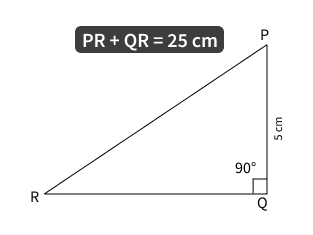# In △PQR, PR + QR = 25cm and PQ = 5cm | Find the value of sin P, cos P, tan P | Trigonometry Numerical

### Question: In △PQR, right angled at Q, PR + QR = 25cm and PQ = 5cm. Determine the values of sin P, cos P and tan P.As shown in the figure of △PQR, let us first write down trigonometric ratios that is asked in the question and using these ratios, by replacing the values of the sides of triangle, we an find the values of sin P, cos P and tan P.

Note: The other approach here can also start by using the given values in question and derive all the values of the sides of triangle. And using the values of the sides of the triangle, find the values of sin P, cos P and tan P by applying appropriate formulas.

Let's start by writing the trigonometric ratios in terms of the sides of △PQR.

\begin{aligned} \sin P &= {\text{opposite side to m} \angle P \over hypotenuse} \\ &= {QR \over PR} \\ \therefore \quad & \boxed{\sin P = {QR \over PR}} \quad --- (1) \end{aligned}

\begin{aligned} \cos P &= {\text{adjacent side to m} \angle P \over hypotenuse} \\ &= {PQ \over PR} \\ \therefore \quad & \boxed{\cos P = {PQ \over PR}} \quad --- (2) \end{aligned}

\begin{aligned} \tan P &= {\text{opposite side to m} \angle P \over \text{adjacent side to m} \angle P} \\ &= {QR \over PQ} \\ \therefore \quad & \boxed{\tan P = {QR \over PQ}} \quad --- (3) \end{aligned}

From equation (1), (2) and (3) we can see that we'll need the values of PQ, QR and PR. Out of these, we already have the value of PQ (5 cm). We also have the value of $PR + QR$. Using these two, let us find the values of QR and PR.

From given data,

\begin{aligned} PR + QR &= 25 \\ \therefore PR &= 25 - QR \quad --- (4) \end{aligned}

Using Pythagoras Theorem on $\triangle PQR$, we get

\begin{aligned} PQ^2 + QR^2 &= PR^2 \\ \therefore 5^2 + QR^2 &= (25 - QR)^2 \quad \quad \text{(replacing value of PR from equation (4))} \\ \therefore 25 + QR^2 &= 25^2 - 2 \sdot 25 \sdot QR + QR^2 \quad \quad \text{(using formula} \ (a - b)^2 = a^2 - 2ab + b^2) \\ \therefore 25 + \cancel{QR^2} &= 25^2 - 2 \sdot 25 \sdot QR + \cancel{QR^2} \quad \quad \text{canceling } QR^2 \text{ from both sides} \\ \therefore 25 &= 625 - 50QR \\ \therefore 50QR &= 625 - 25 \\ \therefore 50QR &= 600 \\ \therefore QR &= {600 \over 50} \\ \therefore \ & \boxed{QR = 12} \quad --- (5) \end{aligned}Putting value of QR in equation (4)

\begin{aligned} PR &= 25 - QR \\ &= 25 - 12 \\ \therefore \ & \boxed{PR = 13} \quad --- (6) \end{aligned}

Now we have the values of PQ, QR and PR as 5, 12 and 13, we can put these values in equation (1), (2) and (3) to get our answers.

## Bonus question #1

The values of the sides of $\triangle PQR$ are 5, 12 and 13. What is the group of values 5, 12, 13 called?

\begin{aligned} \text{From eq. (1)},& \\ \sin P &= {QR \over PR} \\ \therefore \ & \boxed{sin P = {12 \over 13}} \\ \\ \\ \text{From eq. (2)},& \\ \cos P &= {PQ \over PR} \\ \therefore \ & \boxed{cos P = {5 \over 13}} \\ \\ \\ \text{From eq. (3)},& \\ \tan P &= {QR \over PQ} \\ \therefore \ & \boxed{tan P = {12 \over 5}} \\ \end{aligned}

## Bonus question #2

Using a similar approach, can you find the value of sin R, cos R and tan P? Let us know your answers in comments below.